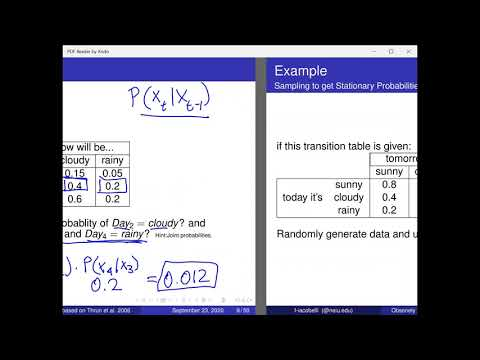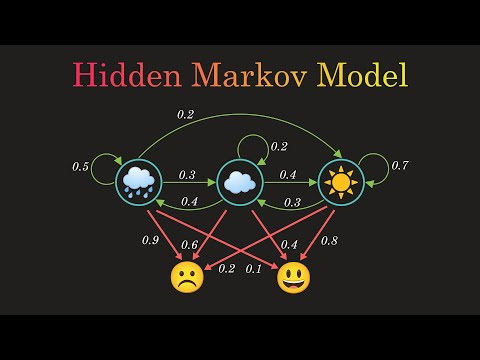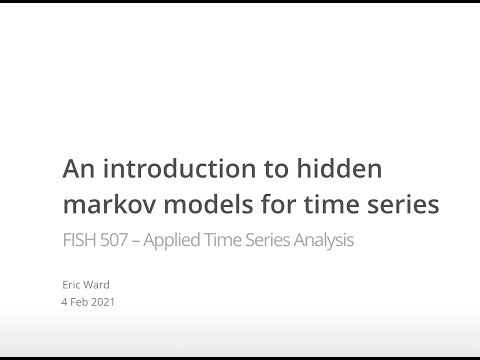# Blog

## What is a hidden Markov model?## What is the use of a time series model?

• A powerful statistical tool for modeling time series data. It is used for analyzing a generative observable sequence that is characterized by some underlying unobservable sequences.

## What will the HMM model look like?

• The HMM model will look like the below picture. We have prior probability P (S)=0.67, Transition probability 0.2, Probability of Bob being Happy given the weather is Sunny P (H|S)=0.8 and Probability of Bob being Grumpy given the weather is Rainy P (G|R)=0.2.